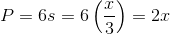## Example Questions

### Example Question #32 : Act Math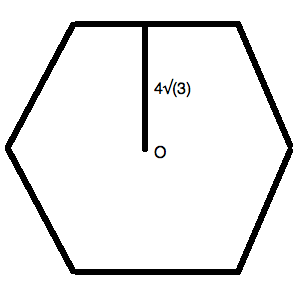The figure above is a regular hexagon.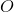is the center of the figure. The line drawn is perpendicular to the side.

What is the perimeter of the figure above?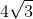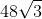Explanation:

You can redraw the figure given to notice the little equilateral triangle that is formed within the hexagon. Since a hexagon can have the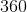degrees of its internal rotation divided up evenly, the central angle isdegrees. The two angles formed with the sides also aredegrees. Thus, you could draw: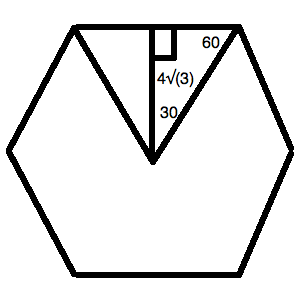Now, theis located on the side that is the same as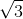on your standard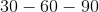triangle. The base of the little triangle formed here ison the standard triangle. Let's call our unknown value.

We know, then, that: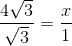Or,Now, this is only half of the size of the hexagon's side. Therefore, the full side length is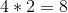.

Since this is a regular hexagon, all of the sides are of equal length.  This means that your total perimeter is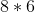or.

### Example Question #33 : Act Math

What is the perimeter of a regular hexagon with an area of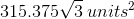?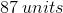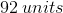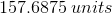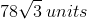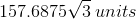Explanation:

The area of a regular hexagon is defined by the equation: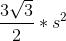, whereis the length of a side.

This is derived from the fact that the regular hexagon can be split up intolittle equilateral triangles, each having an area of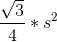To visualize this, consider the drawing: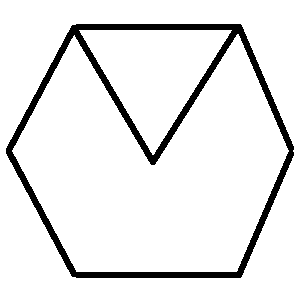Each triangle formed like this will be equilateral.  It is easiest to remember this relationship and memorize the general area equation for equilateral triangles. (It is useful in many venues!)

So, for your data, you know: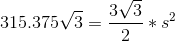Solving for, you get: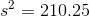This means that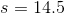Therefore, the perimeter of the figure is equal to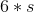or.

### Example Question #34 : Act Math

Find the perimeter of a hexagon with a side length of.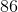Explanation:

There are 6 sides in a hexagon.

Therefore, given a side length of 16, the perimeter is: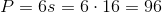### Example Question #35 : Act Math

The side length of a hexagon is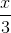.  What is the perimeter?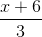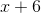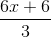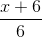Explanation:

Write the formula for the perimeter of a hexagon.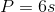Substitute the given length.